Courses

# Shapes And Hybridisation Of Molecules (Part -2) Chemistry Notes | EduRev

## Chemistry : Shapes And Hybridisation Of Molecules (Part -2) Chemistry Notes | EduRev

The document Shapes And Hybridisation Of Molecules (Part -2) Chemistry Notes | EduRev is a part of the Chemistry Course Inorganic Chemistry.
All you need of Chemistry at this link: Chemistry

Molecular orbital Diagrams: Homonuclear Diatomic Molecules up to N2 (i.e. having Z ≤ 14)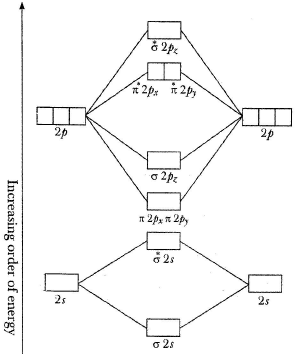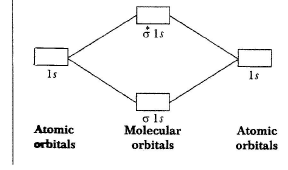Homonuclear diatomic molecule with Z > 14 (O2 onwards)

If the energy differences between 2s and 2p atomic orbitals are small, there is a possibility of mixing of molecular orbitals σ(2s) with σ(2pz) as they are of the same symmetry. Similarly, σ*(2s) with σ*(2pz)  can also mix resulting that σ(2s) and σ*(2s) MOs do not retain the pure s character and the σ(2pz) and σ*(2pz) MOs do not retain the pure p character. In fact, all the four MOs acquire a mixed sp character. Due to this s-p mixing, the energies of all the four orbitals change in such a way that the resulting MOs σ(2s) and σ*(2s), which also contain some p character, become more stable and are thus lowered in energy whereas the MOs σ(2pz) and σ*(2pz) which contain some s character also now become less stable and are thus raised in energy.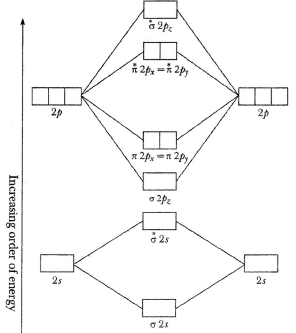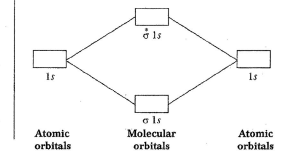Electron Configurations in MO: (Filling of bonding orbitals in order of increasing energy)

• Molecular electron configurations can be written similar to atomic electron configurations.
• Each molecular orbital can hold 2 electrons with opposite spins.
• In these molecular orbitals electrons are filed according toAufbau principle.
• Energy Level Order

From H2 to N2: σls < σ * ls < σ2s < σ * 2s < π2py = π2pz < σ2px < π * 2py = π * 2pz < σ * 2px For O2 & F2: sls < σ * ls < σ2s < σ * 2s < π2px < π2py = π2pz < σ * 2py < π * 2py = π * 2pz = σ * 2p x

• Bond order =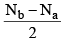N= NO. of electron in bonding Mo’s; Na = No of electron in antibonding Mo’s.

• Bond order (BO) is a measure of net bonding interactions.
• BO must be greater than 0 for a stable molecule to form. If bond order = 0, it means species does not exist.
•  Higher is the BO, stronger is the bond.

Summary of Electronic Configurations of Molecular Orbitals of 1st and 2nd row diatomic Molecules

 Molecule E.C Unpaired e- Bond order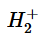σ1s1 1 0.5 H2 σ1s2 0 1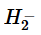σ1s2σ *1s1 1 0.5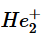σ1s2σ *1s1 1 0.5 He2 σ1s2σ *1s2 0 0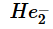σ1s2σ *1s2σ2s1 1 0.5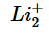σ1s2σ *1s2σ 2s1 1 0.5 Li2 KKσ2s2 0 1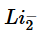KKσ2s2σ * 2s1 1 0.5 Be2 KKσ2s2σ * 2s2 0 0 B2 KKσ2s2σ * 2s2*2p1y π2p1Z 2 1 C2 KKσ2s2σ * 2s2 π2p2y π2p2z 0 2 N2 KKσ2s2σ * 2s2 π2p2y π2p2z σ2p2x 0 3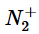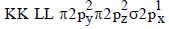1 2.5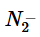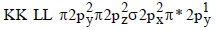1 2.5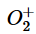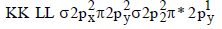1 2.5 O2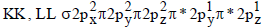2 2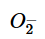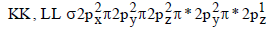1 1.5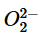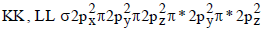0 1.0 F2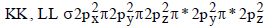0 1 Ne2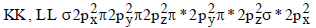0 0

Determination of Magnetism Behaviour: If any molecule has unpaired electron in molecular orbital diagram, then it is paramagnetic. In case of paired electrons the molecule is diamagnetic.

Table. Molecular Orbital Summary of Second Row Diatomic Molecules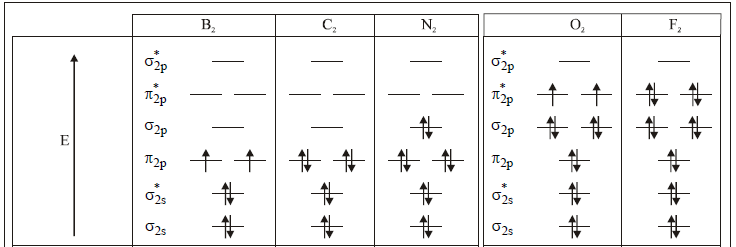Magnetism Paramagnetic Diamagnetic Diamagnetic Paramagnetic Diamagnetic Bond order l 2 3 2 l Observed bond dissociation energy (kJ/mol) 290 620 942 495 154 Observed bond lenglli (pm) 159 131 110 121 143

Illustration: Determine the electron configuration and bond order for the following. if it exists, discuss its magnetism.
Solution: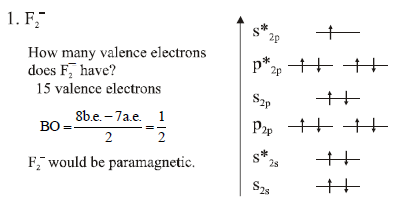Illustration: Determine the electron configuration and bond order for the following. if it exists, discuss its magnetism.
Solution: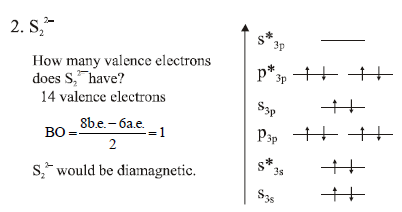Molecular Orbital Treatment for Hetronuclear Diatomic Molecules

• The difference in energy between orbitals on different atoms leads to reduced mixing – The reduced mixing does not imply weaker bonding
• When two atomic orbitals with different energies overlap, the lower molecular orbital is primarily composed of the lower atomic orbital, and vice versa. Moreover, the shift in energies of the two levels is less than if they had the same energy in the atoms.

NO Molecule:

It has 13 electrons therefore its molecular orbital diagram matches to that with Z > 14.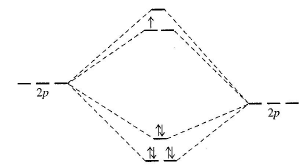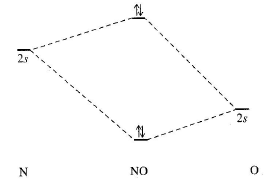Bond order in NO =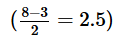NO+: Removal of an electron from NO gives NO+, nitrosonium ion. The electron is removed from π* antibonding MO to given NO+, which resembles the electronic configuration of CO.
Bond order in NO+ =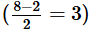Bond order is electronic with N2 and there is shortening of bond length to 106pm

NO: In contrast addition of an electron to π* orbital causes a decrease in bond order and an increase in bond length.
Bond order in NO =   ((8-4)/2) = 2

Out of NO, NO+ and NO, NO has the largest bond and is also paramagnetic.

Note: CN Molecule:

It has 13 electrons thus its molecular orbital diagram is similar to that of Z < 14.
CO Molecule (Coulson Method): The electronic configurations of C and O atoms are 1s22s2p2 and 1s22s22p4, respectively. There are 4 electrons in the outer shell of carbon and 6 electrons in the outer shell of oxygen. Thus, a total of 10 outer electrons are to be accommodated in the molecular orbitals of CO molecule. Because of higher electronegativity of oxygen, its atomic orbitals would be of lower energy than the corresponding atomic orbitals of carbon. Due to this energy difference, the bonding molecular orbitals receive more contribution from atomic orbitals of lower energy, i.e., the atomic orbitals of oxygen and antibonding molecular orbitals would have more characteristics of atomic orbitals of carbon.
The 2s and 2pz atomic orbitals of C atom mix to produce two atomic orbitals of mixed spz character. The higher
energy mixed spz orbital of C atom is closer in energy to its pure 2pz orbital and has major contribution from it whereas the lower energy mixed spz orbital of C is closer in energy to its pure 2s orbital and has major contribution from it. Similarly, 2s and 2pz atomic orbitals of oxygen atom also mix with each other (though not to the same extent to which the orbitals of C atom mix because the energy difference between 2s and 2pz atomic orbitals of oxygen atom is higher than that between the similar atomic orbitals of C atom) to form two atomic orbitals of mixed spz character. The higher energy mixed spz orbital of O is very close in energy to its pure 2pz orbital and has contribution mostly from 2pz orbital whereas the lower energy mixed spz orbital, being very close in energy to pure 2s orbital of oxygen atom, has contribution mostly from 2s orbital of oxygen atom.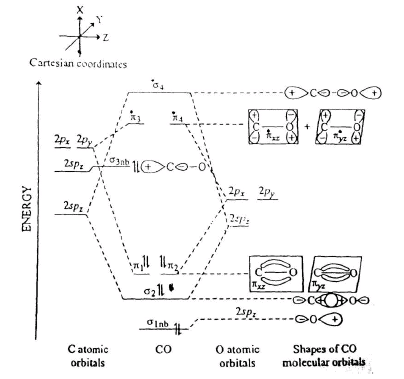Since the orbital energies are lower for more electronegative O atom compared to the energies of similar orbitals of C atom, the lower energy mixed spz orbital of C atom is comparable in energy to the higher energy spz orbital of O atom, as shown in MOEL diagram of CO. These two atomic orbitals of comparable energy and of same symmetry combine to give s bonding and antibonding molecular orbitals. The lower energy spz, orbital on O and the higher energy sp2 orbital on C atom are so far apart in energy that they hardly influence each other with the result that their energies remain almost unchanged.
In other words, these orbitals remain almost non bonding, the non bonding spz orbital on C(σ3nb in the figure) will have more characteristics of 2pz orbital of C atom and the non bonding spz orbital on O atom C(σ3nb in the figure) will have more characteristics of 2s orbital of O atom. The degenerate set of 2px2py orbitals of C and the similar degenerate set of 2px2py orbitals of oxygen are of p symmetry to form a set of degenerate π12 bonding molecular orbitals and another set of degenerate antibonding molecular orbitals. The electronic configuration of CO molecule is, therefore,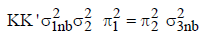and the bond order of CO molecule is obviously 3 (due to two σ bonding and four p bonding) electrons.
The electrons in the non bonding orbitals do not contribute towards bonding.
Since σ3nb is the highest placed occupied non bonding orbital localised on C atom, its electron pair can easily be donated for forming dative (or coordinate) bond.
NOTE: Bond order of CO molecule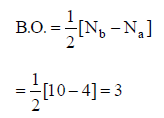Bond order of CO+ ion

Bond order remains same as that of CO as electron is withdrawn from non bonding orbital rather than from anti bonding orbital.
Hydrogen Flouride Molecule, HF

The two 2s and 2pz of F, being of the same symmetry, can mix with each other. However, the extent of mixing of 2s and 2pz orbitals will be small because the energy gap between in these two orbitals in high.
The 1s orbital of hydrogen atom is more comparable in energy to the higher energy mixed spz orbital (which is practically 2pz orbital) of F. The two combine to give σ1 bonding and σ3* antibonding molecular orbitals. The lower energy mixed spz orbital of F cannot effectively combine with 1s orbital of H atom due to large energy difference between this mixed orbital and 1s orbital of H atom and, therefore, remain almost non bonding (σ2nb) orbital. The molecular orbital energy level diagram of HF is shown in following figure.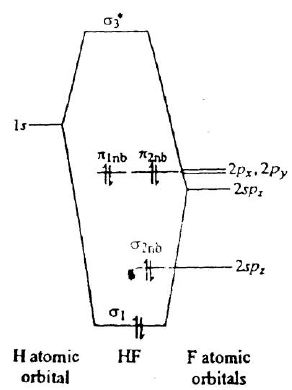The electronic configuration of HF molecule is thus,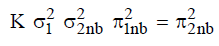and the bond order is obviously 1 since only two electrons occupy the bonding molecular orbital σ1.

Term Symbols for Diatomic Molecules

Term symbols are used to designate electronic energy levels which arise out of an electronic configuration because of electrostatic repulsions amongst the electrons of a molecule. These terms are very helpful in understanding electronic spectra of molecules.

The term symbols employed for diatomic molecules are written in the following manner:

(i) The symbols ∑ , π and Δ are used to represent values 0, 1 and 2 respectively for |ML| or ∑ mL is 0,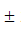1 and2 for an electron in σ, π and δ molecular orbitals, respectively.

(ii) Since all homonuclear diatomic molecules have a centre of symmetry in the middle of their nuclear axis, the overall symmetry to inversion is indicated by u or g and is written as a right subscript of ∑ , π,  Δ.
(iii) The electron spin multiplicity in indicated by 2S + 1 as the left superscript of ∑ , π and Δ (S is the total spin of electrons in the system).
iv) The term symbol is written as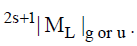For example, consider a H2 molecule whose ground state electronic configuration is σ2(1s).
In this case, S(i.e. s1 + s2) is 0 and |ML|i.e.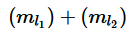is also 0. The σ1s molecular orbital is symmetric with respect to the centre of inversion located in the middle of the nuclear axis, Therefore the term symbol for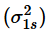should be g × g = g type. Hence, the term symbol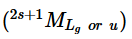for H2 molecule in its ground state, is 1g
Consider now hydrogen ion, H+2 its ground state electronic configuration is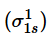so that S = 1/2 and 2S + 1 = 2. Since |ML| = 0, hence the term symbol for its ground state is 2g .
Let us now work out the term symbols for O2 molecule. The ground state electronic configuration of O2 is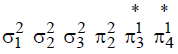. The electrons in the filled sigma molecular orbitals and the completely filled degenerate set of pi orbitals contribute nothing to the overall component of angular momentum ML along the nuclear axis. Since both the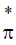molecular orbitals are symmetric with respect to the centre of symmetry of the oxygen molecule, the term symbol will be g × g = g type.
Let us first consider the case when both the unpaired electrons inmolecular orbitals have opposite spins. The ml for each electron inmolecular orbital will be +1 or -1 so that |ML| = 0 or 2 and 2s + 1 = 1 (because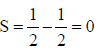). The term symbols which arise from this situation are 1g and 1Δg .
Now consider the case when the spins of both the electrons are in the same direction so that 1 1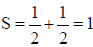and the spin multiplicity is 3. Since spins of both the electrons in π* orbitals are in the same direction, their ml values have to be different (Paulis exclusion principle). That is, ml for one electron is +1 and for the other electron it is -1 (The + and - signs indicate whether the component of that the term is 3g .
Out of 3g , 3g and 1Δg the first term, i.e., 3g , having maximum multiplicity will evidently represent the ground state term of the molecule.
Terms for Li2, Be2, N2, F2, F2+ molecules try yourself.
The term symbols for heteronuclear diatomic molecules are derived in a similar manner. Since a heteronuclear diatomic molecule has no centre of symmetry, we do not write the subscript g or u in the term symbols of such molecules.
The ∑ term are sometimes further codified by writting + or - as a right superscript depending upon whether the system is symmetric or asymmetric with respect to a plane of symmetry passing through the nuclear axis.

Offer running on EduRev: Apply code STAYHOME200 to get INR 200 off on our premium plan EduRev Infinity!

## Inorganic Chemistry

33 videos|59 docs|16 tests

,

,

,

,

,

,

,

,

,

,

,

,

,

,

,

,

,

,

,

,

,

;